共有1002人关注过本帖树形打印复制链接

# 主题：[求助]等级划分的代码怎么修改才能达到想要的效果？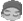1楼 | 信息 | 搜索 | 邮箱 | 主页 | UC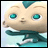加好友发短信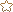[求助]等级划分的代码怎么修改才能达到想要的效果？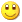Post By：2019/1/21 12:42:00 [只看该作者]

 下载信息  [文件大小：   下载次数： ]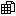点击浏览该文件:等级.egd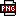此主题相关图片如下：qq图片20190121123758.png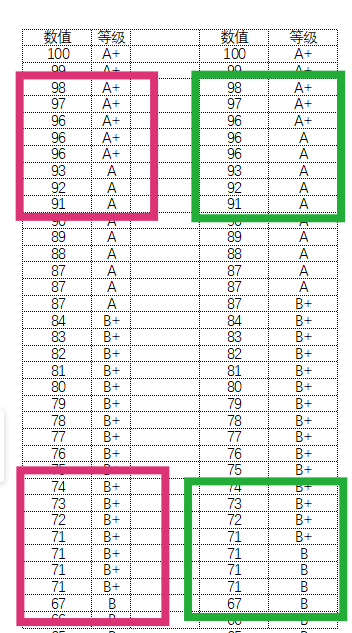此主题相关图片如下：qq图片20190121124419.png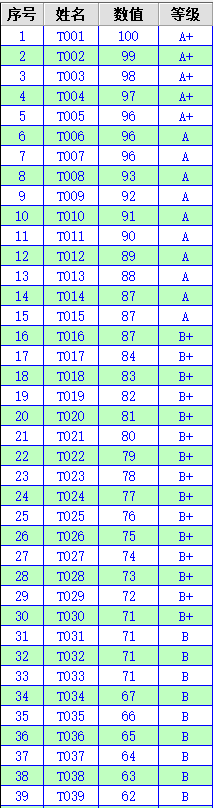[此贴子已经被作者于2019/1/21 12:53:03编辑过]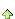iyplx2425
2楼 | 信息 | 搜索 | 邮箱 | 主页 | UC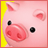加好友发短信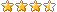Post By：2019/1/21 14:32:00 [只看该作者]

 [等级]刷新公式：IF(Row <= [数值] * 0.05,"A+",IF(Row <= [数值] * 0.15,"A",IF(Row <= [数值] * 0.3,"B+",IF(Row <= [数值] * 0.5,"B",IF(Row <= [数值] * 0.75,"C+",IF(Row <= [数值] * 0.75,"C",IF(Row <= [数值] * 0.85,"D+",IF(Row <= [数值] * 0.95,"D","E"))))))))按F6即可。3楼 | 信息 | 搜索 | 邮箱 | 主页 | UC加好友发短信Post By：2019/1/21 17:49:00 [只看该作者]

 以下是引用iyplx2425在2019/1/21 14:32:00的发言：[等级]刷新公式：IF(Row <= [数值] * 0.05,"A+",IF(Row <= [数值] * 0.15,"A",IF(Row <= [数值] * 0.3,"B+",IF(Row <= [数值] * 0.5,"B",IF(Row <= [数值] * 0.75,"C+",IF(Row <= [数值] * 0.75,"C",IF(Row <= [数值] * 0.85,"D+",IF(Row <= [数值] * 0.95,"D","E")))))))) 按F6即可。此主题相关图片如下：qq图片20190121174658.png多谢大师帮忙，可测试的效果如图所示，没有达到想要的效果，是哪出了问题呢   下图是用电子表格划出的等级 ，这个才是我想要的效果，哪位大师帮忙看看，谢谢。此主题相关图片如下：qq图片20190121202424.png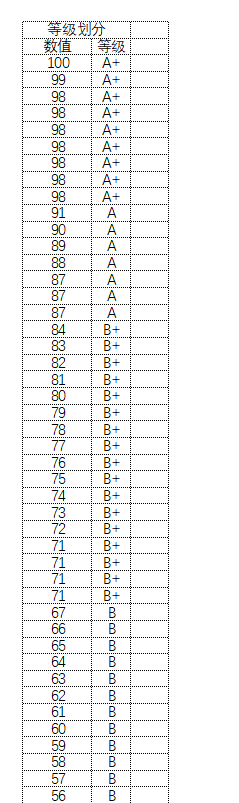[此贴子已经被作者于2019/1/21 20:26:00编辑过]4楼 | 信息 | 搜索 | 邮箱 | 主页 | UC加好友发短信Post By：2019/1/22 9:08:00 [只看该作者]此主题相关图片如下：qq图片20190121174658.png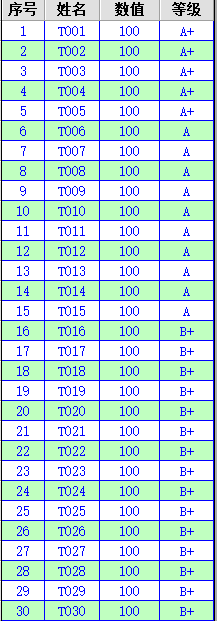此主题相关图片如下：qq图片20190121202424.png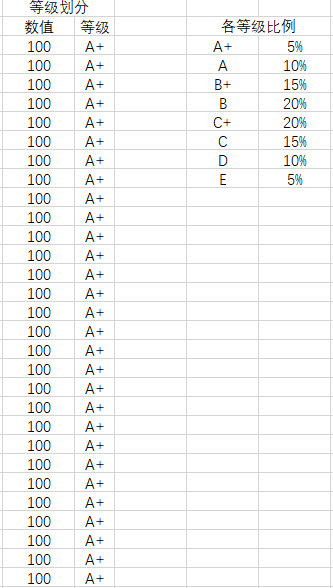一样的等级比例标准，在数值相同的情况下，易表为什么划分了不同的等级、而EXcel只划分一个等级，有没有办法也让易表在数值相同的情况下只划分一个等级呢lyjzyq
5楼 | 信息 | 搜索 | 邮箱 | 主页 | UC加好友发短信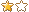Post By：2019/1/22 14:51:00 [只看该作者]

SortCol("数值",2) And EvalFor(GridName,"等级","If([数值] >95,""A+"", If([数值] >85,""A"",If([数值] >70,""B+"",If([数值] >50,""B"",If([数值] >30,""C+"", If([数值] >15,""C"",If([数值] >5,""D"",""E"")))))))")
 下载信息  [文件大小：   下载次数： ]点击浏览该文件:等级.egd6楼 | 信息 | 搜索 | 邮箱 | 主页 | UC加好友发短信Post By：2019/1/22 15:54:00 [只看该作者]

SortCol("数值",2) And EvalFor(GridName,"等级","If([数值] >95,""A+"", If([数值] >85,""A"",If([数值] >70,""B+"",If([数值] >50,""B"",If([数值] >30,""C+"", If([数值] >15,""C"",If([数值] >5,""D"",""E"")))))))")
 下载信息  [文件大小：4.6 KB  下载次数：0]点击浏览该文件:等级.egd7楼 | 信息 | 搜索 | 邮箱 | 主页 | UC加好友发短信Post By：2019/1/22 16:38:00 [只看该作者]此主题相关图片如下：qq图片20190122163549.png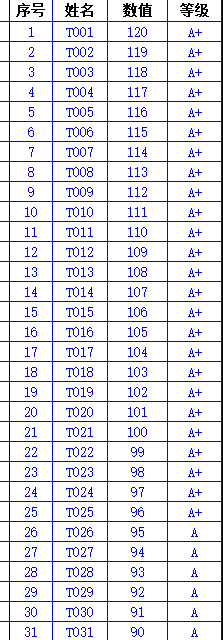A+ 5% A 10% B+ 15% B 20% C+ 20% C 15% D 10% E 5%

[此贴子已经被作者于2019/1/22 17:20:01编辑过]lyjzyq
8楼 | 信息 | 搜索 | 邮箱 | 主页 | UC加好友发短信Post By：2019/1/23 20:34:00 [只看该作者]

 下载信息  [文件大小：   下载次数： ]点击浏览该文件:等级1.egd9楼 | 信息 | 搜索 | 邮箱 | 主页 | UC加好友发短信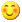Post By：2019/1/24 18:15:00 [只看该作者]

 下载信息  [文件大小：4.7 KB  下载次数：1]点击浏览该文件:等级1.egdiyplx2425
10楼 | 信息 | 搜索 | 邮箱 | 主页 | UC加好友发短信Post By：2019/1/27 10:37:00 [只看该作者]

 [等级]的刷新公式为：IF(Row <= Rows * 0.05,"A+",IF(Row <= Rows * 0.15,"A",IF(Row <= Rows * 0.3,"B+",IF(Row <= Rows * 0.5,"B",IF(Row <= Rows * 0.7,"C+",IF(Row <= Rows * 0.85,"C",IF(Row <= Rows * 0.95,"D","E")))))))，好像就可以了啊。总数 12 1 2 下一页